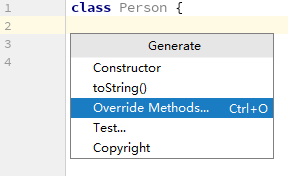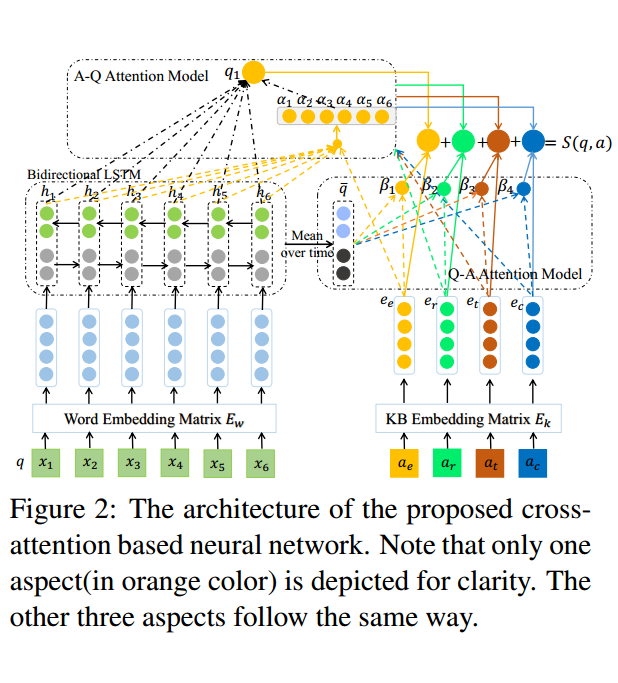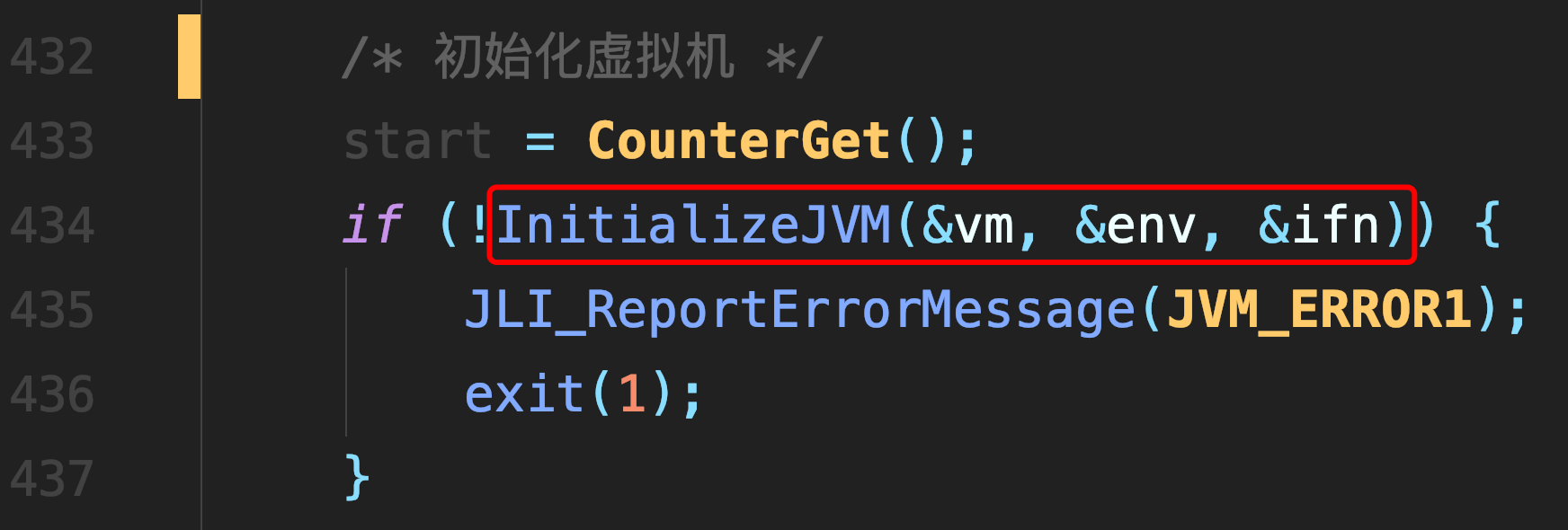# 梯度下降法

### 梯度下降法

• 梯度

• 方向导数：定义 $f$是定义于 $R^n$ 中某区域D上的函数，点 $P_0 \in D$

$l$为一给定的非零向量， $P$为一动点，向量 $P_0P$ 与l的方向始终一致。
$如果极限\lim_{\|P_0P\|\to0}\frac{f(P)-f(P_0)}{\|\overrightarrow{PP_0}\|}存在$
则称此极限为函数 $f$ $P_0$处沿l方向的方向导数，记作 $\frac{\partial f} {\partial l}$

• 梯度定义如下
$grandf(x_0,x_1,...,x_n)=\{\frac{\partial f}{\partial x_0},\frac{\partial f}{\partial x_1}, ...\frac{\partial f}{\partial x_n} \}$
梯度是一个向量，方向与最大方向导数方向一致，模是方向导数的最大值，所以沿着

梯度方向，函数值增长最快，另一方面看，就是沿着反梯度方向，函数值下降最

证明梯度方向是最大导数方向，且模是方向导数的最大值

• 具体的例子
$假设函数模型为 h(\theta)=\theta_0+\theta_1x_1+\theta_2x_2+...+\theta_nx_n=\sum_{i=0}^{n}\theta_ix_i$

$则损失函数为 J(\theta)=\frac{1}{2m}\sum_{i=1}^{m}(h_\theta(x^i)-y^i)^2, m代表样本数量,多乘以\frac{1}{2}是为了让偏导结果更简洁$

$我们的目标: \mathop{min}_{\theta}J(\theta)$

$对每个\theta_j求偏导 \frac{\partial J(\theta)}{\partial \theta_j}=\frac{1}{m}\sum\limits_{i=1}^m(h_\theta(x^i)-y^i)x_j^i$

$之后按梯度的负方向更新每一个\theta_j, \theta_j=\theta_j-\alpha\frac{\partial J(\theta)}{\partial_j}, 其中\alpha为迭代的步长， 迭代直到收敛$

##### 热门推荐
•浏览(654)
•浏览(563)
•浏览(547)
•浏览(480)
•浏览(424)
•浏览(419)
•浏览(381)
•浏览(366)
•浏览(362)
•浏览(344)